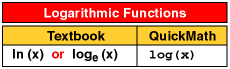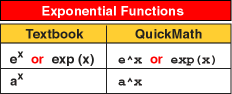Home | About | Contact | Disclaimer | Help

Algebra

Equations

Inequalities

Graphs

Numbers

Calculus

Matrices

Tutorials

### Help: Logarithmic and exponential functions

Here is a complete list of logarithmic and exponential functions accepted by QuickMath. The tables show the usual form in which the functions appear in textbooks, along with the form accepted by QuickMath. In most cases, the QuickMath version is identical to the textbook version.

If there is a function missing which you would like see added to those supported by QuickMath, just send your suggestion to comments@quickmath.com.# PROPERTIES OF LOGARITHMIC FUNCTIONS

OBJECTIVES

1. Convert Exponentials to Logarithmic Forms and Vice Versa
2. Evaluate Logarithms Base 10 and Base e
3. Use the Properties of Logarithms
4. Use the Change-of-Base Formula
5. Solve Applied Problems

In the previous section we learned that the graph of the inverse function f-1 1 is a reflection of the graph of f across the line y = x. Exponential functions are one-to-one, so they have inverses. have inverses. Consequently, if we graph the function .f (x) =bx and reflect its graph across the line y = x, the result is the graph of f-1. This new function is given the name logarithmic function with base b, and it is written asFor example, as Figure 1 shows, the graph of f-1(x) = log2, is the reflection of the graph of f (x) = 2x  across the line y = x. Since logarithms, in a sense, evolve as inversesof exponential functions,  algebra of logarithms is derived from the algebra of exponents, as we shall see.

Converting Exponentials to Logarithmic Forms and Vice Versa

A logarithm is actually an exponent. For instance, consider the expression  49 = 7x
We refer to:

2 as the logarithm of 49 with base 7,

and we write:

log7 49 = 2.

The logarithm value 2 is the exponent to which 7 is raised to get 49. In general, we have the following logarithm definition:

Let b > 0 and b<> 1. The logarithm of x with base b, which is represented by y, is defined by

y = logb x if and only if  x = by for every x > 0 and for every real number y

In words, this definition says that the logarithm y is the exponent to which b is raised to get x

The two equations in the above definition are equivalent and as such can be used interchangeably. The first equation is in logarithmic form and the second is in exponential form. The diagram below is helpful when changing from one form to the other:Base of logarithm is the same as exponent base

It is important to observe that logb x is an exponent. For instance, the numbers in the right column of Table 1 are the logarithms (base 2) of the numbers in the left column.

Converting Exponentials to Logarithmic

Convert each exponential form equation to logarithmic form,The equivalencies are listed in the following table.Converting Logarithmic to Exponentials

Convert each logarithmic form to an equivalent exponential form.The following table lists the equivalency of each logarithmic form.At times, we can find the numerical value of a logarithm by converting to exponential form and then using the one-to-one property of exponents, as the next example illustrates.

Evaluating Logarithms

Evaluate each logarithm.In each case we let u equal the given expression, and write the logarithmic equation in its equivalent exponential form and then solve the resulting equation for u as shown in the following table.We use the equivalency between the logarithmic and exponential forms to solve certain equations involving logarithms, as the next example shows.

Solving Logarithmic Equations

Solve each logarithmic equation for x.First we rewrite the given equation in exponential form and then solve the resulting equation.Evaluating Logarithms - Base 10 and  Base e

The base of a logarithmic function can be any positive number except 1. However, the two bases that are most widely used are l0 and e.A logarithm with base 10 is called a common logarithm. Its value at x is denoted by log x, that is,A logarithm with base e is called a natural logarithm, and its value at x is denoted by In x, that is,Consequently:Sometimes we can use the definition of logarithms to evaluate common and natural logarithms easily. For instance: